×

Search anything:

# expr command in Linux

#### Linux Software EngineeringIn Linux, the expr command evaluates an expression passed to it and returns the corresponding value. We usually use it for basic arithmetic such as addition, subtraction, modulo operations, division, multiplication. We also use expr command to evaluate expressions.

1. Introduction.
2. Syntax.
3. Basic arithmetic.
4. Comparisons.
5. String operations.
6. Summary.
7. References.

## Introduction.

The expr command, as the name suggests, is used to evaluate expressions in the bash terminal and also in shell scripts. In the following sections, we look at basic expr commands in Linux.

## Syntax.

We write expr commands using the following format:

``````\$ expr [EXPRESSION]
``````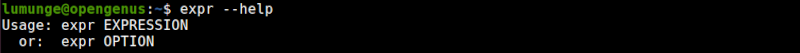## Basic arithmetic.

As mentioned earlier, we use the expr command for basic arithmetic on the Linux terminal.

We can perform additions, subtractions, multiplications, modulo operations and divisions.

To add to numbers we write:

``````\$ expr num1 + num2
``````

To subtract them we write:

``````\$ expr num1 - num2
``````

To divide them we write:

``````\$ expr num1 / num2
``````

To multiply them, we have to escape the * operator as follows:

``````\$ expr num1 \* num2
``````

To get the modulus of two numbers we write:

``````\$ expr num1 % num2
``````Apart from performing arithmetic operations on the bash terminal, we can also perform operations on variables within a shell script:

``````echo "Enter two values: "
echo "Their sum is: `expr \$a + \$b`"
``````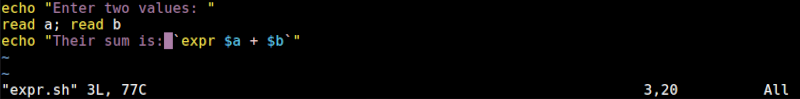The output: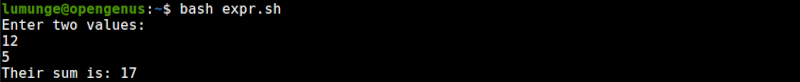We can also increment and decrement variables, for example, to increment a variable's value by 3 we write:

``````\$ expr \$x + 3
``````

And to decrement it by 4 we write:

``````\$ expr \$x - 4
``````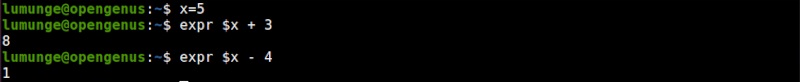## Comparison.

We can evaluate two expressions to check if they are similar or not, or one is less than or greater than or one is not equal to or is equal to the other.

For example to check if two strings match we write:

``````# equality
\$ expr \$string1 = \$string2

# not equal
\$expr \$string1 \!= \$string2
``````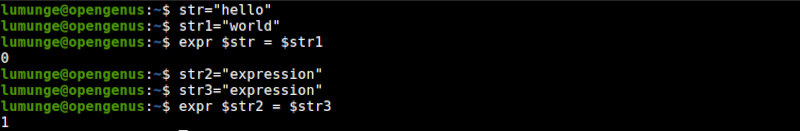Another example;To check if one number is less than or greater than another we write:

``````\$ expr \$num1 \> \$num2

\$ expr \$num1 \< \$num2
``````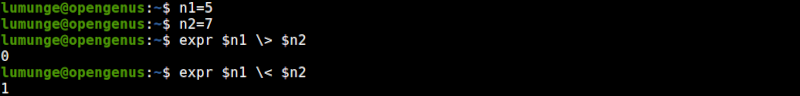We can also evaluate boolean expressions, for example:

``````\$ expr 4  "<"  5  "&"  6  ">="  10
``````

The above returns false(0) since 4 is less than 5 but 6 is not greater than or equal to 10.
This is an example of a logical AND using expr command.

Using the logical OR we can write:

``````\$ expr 4  "<"  5  "|"  6  ">="  10
``````

Here the second expression is false, however, if any is true then the expression is true.

## String operations.

We also use expr to find the length of strings. The basic syntax is as follows:

``````\$ expr length "The length of a string"
``````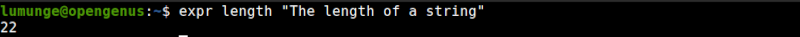We can also find substrings - strings within strings. For example, to get a substring starting from index 4 and is four characters long, we write:

``````\$ expr substr \$string 4 4
``````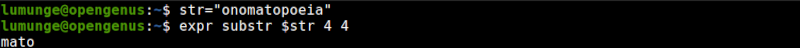This command is also handy in matching the number of characters between two strings, for example:

``````\$ str1=Javascript
\$ str2=Java
\$ expr \$str1 : \$str2
``````In the above example, the result is 4 meaning only four characters are common to both strings.

To find the position of a specific character in a string we write:

``````\$ expr index \$string [character]
``````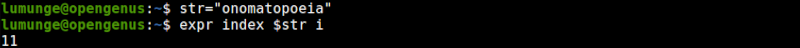Here we try to return the position of the character x in string.

## Summary.

We use the expr command to evaluate expressions in the bash terminal. We use it for basic operations and evaluation of regular expressions. We also use it to perform string operations such as returning the length of a string and getting substrings from a string.